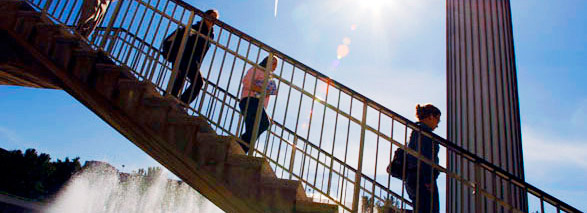## Algebra, Combinatorics and Number Theory Department of Mathematics and Statistics

Our research group in Algebra, Combinatorics and Number Theory includes specialists in algebraic combinatorics, Lie algebras, algebraic number theory, and commutative algebra. Their work includes applications to mathematical physics, representation theory, algebraic geometry, and topological data analysis. There is significant interaction within this research group, and between them and the topologists. Here are their personal research statements.

### Cristian Lenart"My research is concerned with the development of new computational models, based on combinatorial structures, for various areas in algebra and geometry. This type of work is at the forefront of current mathematical research, being related to developments within mathematics itself (the current emphasis on computation), to the advent of computers, as well as to applications to theoretical physics.

"I use combinatorial structures/methods in representation theory (a fundamental tool for studying group symmetry), and in the study of certain algebraic varieties. I am particularly interested in flag varieties and Schubert varieties, which have many applications to representation theory and enumerative problems in geometry (for instance, counting the lines or planes satisfying a number of generic intersection conditions); these varieties are also a very useful testbed for larger classes of varieties, and are related to modern areas such as quantum cohomology and quantum K-theory.

"My work in representation theory is related to several recent developments, such as those involving affine Lie algebras, quantum groups, and Macdonald polynomials. Computer experiments play an important role in my research."

### Antun Milas"My work focuses on vertex operator algebras. These are relatively new algebraic structures playing important role in conformal field theory and other areas of mathematics and physics. The notion of vertex algebra was introduced by R. Borcherds in mid-80s, and was later used by I. Frenkel, J. Lepowsky and A. Meurman for construction of the largest sporadic finite simple group, the Monster. Most recently, vertex algebras, or more precisely chiral algebras, have proven useful in Beilinson--Drinfeld's geometric version of the Langlands correspondence. There are also applications of these concepts in topology.

" I am mainly interested in algebraic aspects of two-dimensional conformal field theory, through studies of various (categories of) representations of vertex algebras and quantum groups. My current interests include representation theory of irrational vertex algebras and connections to modular-like functions. The motivational dream is to formulate and prove a Verlinde-type formula for general vertex algebras."

### Anupam Srivastav"I work in algebraic number theory, with a particular interest in Galois module theory. This interest is motivated by relationships between algebro-arithmetic invariants and analytic invariants associated with Galois extension of number fields. If M/K is a Galois extension of number fields with Galois group G, an algebro-arithmetic invariant is associated to the H-module S in a classgroup of H, where S is the ring of integers of M and H is the associated order of S in the group algebra KG. Analytic invariants arise from L-functions (or p-adic L-functions) associated with number fields K and M.

"Much of my work has been about such algebro-arithmetic invariants that come from a subgroup of the classgroup of H, called the Swan subgroup. I am also interested in the theory of cyclotomic fields and the arithmetic of elliptic curves."

### Alexandre Tchernev"My research is in Commutative Algebra --- an area of mathematics that studies the structure and properties of commutative rings, and of modules over commutative rings. My current research is focused on investigating the properties of resolutions of modules. A resolution of a module, especially a so-called ``minimal resolution", is an algebraic structure that encodes essential algebraic properties of a module into a sequence of matrices. Once the properties of a resolution of a module are well understood, one can then utilize the powerful already available tools of homological algebra to better understand the algebraic properties of the module. It is thus of essential interest to develop techniques to better understand and, if possible, explicitly construct minimal resolutions of modules.

"This is what my graduate students and I are working on. Examples of important classes of modules where this work has been recently successful are monomial ideals, and multigraded modules over polynomial rings. These modules are of great interest, for instance, in combinatorics, symbolic computation, algebraic geometry, and topological data analysis."

### Changlong Zhong"I am interested in algebraic geometry and representation theory. More precisely, I am interested in flag varieties, their cohomology groups, and their relationship with geometric representation theory and algebraic combinatorics. Flag varieties come with combinatorial structure and actions of algebraic groups, so their cohomology groups have nice properties. These properties are closely related to representations of certain groups (e.g., finite groups, Coxeter groups and algebraic groups). They also provide information about the flag varieties themselves, for example, the subvarieties and their intersections.

"It was recently found that this study of flag varieties using cohomology groups is parametrized by one dimensional commutative formal group laws, and classical work is only for the additive and multiplicative formal group laws. I am interested in the study of Hecke algebras and Schubert Calculus in case of more general formal group laws, for example, the elliptic formal group laws."